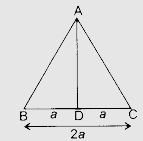# ABC is an equilateral triangle of side $2a$. Find each of its altitudes.

Given:

ABC is an equilateral triangle of side $2a$.

To do:

We have to find each of its altitudes.

Solution:In $∆ABC$,

$AB = BC = AC = 2a$ and $AD \perp BC$

$BD = \frac{1}{2}(2a)$

$= a$

$BD=DC=a$

In right angled triangle $ABD$,

$AD^2 + BD^2 = AB^2$

$AD^2 = AB^2 - BD^2$

$= (2a)^2 - (a)^2$

$= 4a^2 - a^2$

$= 3a^2$

$\Rightarrow AD = \sqrt3a$

Therefore,

Each altitude of the triangle is equal to $\sqrt3a$.

Updated on: 10-Oct-2022

34 Views